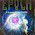## Wednesday, February 25, 2015

### The beauty and mysteries of imaginary time and temperature

Yesterday, at UQ Robert Mann gave a nice Quantum Science seminar, "Hot and Cold Accelerating Detectors".

It concerned the Unruh effect: suppose one observer is constantly accelerating relative to another. Then, what is a quantum vacuum (for a free boson or fermion field) to one observer is a thermally populated state to the other.

Specifically, if one considers a field with wave vector k and energy Ω k. Then the expectation of the number operator is,
$\begin{array}{}\text{(5)}& ⟨0|{b}_{k}^{†}{b}_{k}|0⟩=\left({e}^{2\pi {\mathrm{\Omega }}_{k}/g}-1{\right)}^{-1},\end{array}$
which corresponds to a Bose distribution with a temperature given by
$\begin{array}{}& {T}_{\mathrm{U}\mathrm{n}\mathrm{r}\mathrm{u}\mathrm{h}}=\frac{g}{2\pi }=\frac{\hslash g}{2\pi c{k}_{B}}\end{array}$
where g is the constant acceleration. Note that this formula involves relativity (c), quantum physics (hbar), and statistical mechanics (kB).

I feel there is something rather profound going on here.
Without doing the calculation, it is perhaps not totally surprising that the accelerated observer sees a non-trivial occupation of excited states of the quantum field.
However, what is rather surprising to me is that the state occupation numbers has to be that associated with thermodynamic equilibrium.   Why not some other distribution? And that this holds for both fermions and bosons.
After all, you are starting purely with relativity [and the mathematics of Rindler co-ordinates] and quantum field theory and you are ending up with quantum statistical mechanics.

Is the thermal distribution just a "mathematical accident" because cosh functions are appearing in the Rindler co-ordinates, Bogoliubov transformation of the quantum field, and in thermal distribution?

The Scholarpedia page is helpful, stating this

can also be understood as a manifestation of the general relationship between temperature and imaginary time in quantum statistical mechanics (KMS theory). When t and τ are extended as complex variables, iτ is revealed as an angular variable in the (it,z) plane. The periodicity determined by g, the acceleration, is the only one that makes functions of τ be analytic in t. That period corresponds, under the KMS theory, to the reciprocal of the Unruh temperature (Dowker, 1978; Christensen and Duff, 1978; Sewell, 1982; Bell et al., 1985; Fulling and Ruijsenaars, 1987).''

I welcome any further elucidations on this rich and subtle issue.

#### 4 comments:

1.Can you put was seems to be a fascinating subject in language that the lay person can understand.

2.Hi Ross, your exponential is missing brackets, or something. And from the lower equations, it follows that
ℏc k B = 1
which is incorrect, by order of magnitude analysis. Nice topic, though.
~ Tinos

3.Hi Ross, as a relativist who follows you're blog with pleasure is nice to see you interested in the Unruh effect.

There are two different concepts which enter here. The first regards the fact that non-inertial observers observe the usual Minkowski vacuum as a state with particles. This is just a consequence of Bogoliubov transformation, as you noted. In any case I have given a somewhat detailed account of this in stackexchange here http://physics.stackexchange.com/questions/165777/what-is-the-essence-of-the-unruh-effect

The second, the one I take you found more curious, is that according to accelerated observers the Minkowski vacuum is a thermal (KMS) state. An interpretation which I prefer compared to imaginary time (Matsubara formalism) is the Araki-Woods (also called Thermofield dynamics, the most elementary discussion I know is in Ashok Das' Finite Temperature Field Theory).

The idea is simple. Suppose you want to treat a KMS state as a vector is the Hilbert state (this is called purification of KMS states). In the 60s Araki and Woods found out that if you double the size of your Hilbert state then a pure vector can have the same expectation values as a thermal density matrix in the original theory.

This is exactly what occurs in Unruh effect (and Hawking effect for that matter). Since an accelerated observer sees an horizon it does not have access to half of the field modes (the ones contained in the other Rindler wedge). Therefore causal ignorance of what happens in the other Rindler wedge (which has the "same" size as yours) is translated into maximum ignorance regarding correlations in the state you observe in your own Rindler wedge, giving you a thermal state.

But one must remember that although the state is KMS it does not imply that an accelerated observer sees the same physics as an inertial one in a thermal bath. An inertial thermal bath is isotropic and the accelerated nature of the observer picks a preferential direction in space. This distinction is behind the fact that although the Unruh effect atributes a temperature to the vaccum, an accelerated observer cannot see witness a phase transition, no matter how high the temperature is.

1.Cesar,

Thanks for the helpful comment.
I am slowly seeing that the thermal distribution is not just a "mathematical accident" but is due to physics. In particular, you state:
"causal ignorance of what happens in the other Rindler wedge .... is translated into maximum ignorance regarding correlations in the state you observe in your own Rindler wedge, giving you a thermal state".

This highlights that thermal states maximise "ignorance".